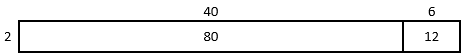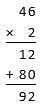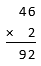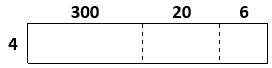Match Fishtank is now Fishtank Learning!

# Multiplication and Division of Whole Numbers

## Objective

Multiply two-digit, three-digit, and four-digit numbers by one-digit numbers.

## Common Core Standards

### Core Standards

?

• 5.NBT.B.5 — Fluently multiply multi-digit whole numbers using the standard algorithm.

?

• 4.NBT.B.4

• 4.NBT.B.5

• 5.NBT.A.1

• 5.NBT.A.2

## Criteria for Success

?

1. Compute two-digit by one-digit, three-digit by one-digit, and four-digit by one-digit products using the area model, the partial products algorithm, and the standard algorithm.
2. Relate the area model, the partial products algorithm, and the standard algorithm.
3. Round to estimate two-digit by one-digit, three-digit by one-digit, and four-digit by one-digit products.

## Tips for Teachers

?

• Students have seen the area model, the partial products algorithm, and the standard algorithm strategies for all computational types presented in this lesson in Grade 4. The intention of this lesson it to solidify these strategies, and particularly the standard algorithm, with these cases before moving on to more cognitively complex cases in later lessons.
• Throughout this topic, it is important to preserve place value when talking about products of multi-digit numbers. For example, when discussing the computation 1,768 x 4, the partial product of the 6 digit and the 4 digit should be referenced as “six tens times four” or “sixty times four” rather than “six times four.”

#### Fishtank Plus

• Problem Set
• Student Handout Editor
• Vocabulary Package

?

### Problem 1

Here is one way to find the area of a rectangle that is 43 units by 2 units.You might be familiar with different ways to write multiplication calculations. Here are two ways to calculate 46 x 2.Calculation A                     Calculation B

1. In Calculation A, how are each of the partial products obtained? For instance, where does the 12 come from?
2. In Calculation B, how is 92 obtained?
3. Look at the area model. How are the partial products in Calculation A and the 92 in Calculation B related to the numbers in the area model?

#### References

Open Up Resources Grade 6, Unit 5, Lesson 7, Teacher Version7.2: Connecting Area Diagrams to Calculations with Whole Numbers

Modified by Fishtank Learning, Inc.

### Problem 2

1. The area model below represents 326 x 4.1. Before calculating, estimate the product 326 x 4.
2. Label each region of the area model with its respective area.
3. Find the value of 326 x 4. Show your reasoning.

2. Go through a similar process for 234 x 8.

#### References

Open Up Resources Grade 6, Unit 5, Lesson 7, Teacher Version7.3: Connecting Area Diagrams to Calculations with Decimals

Modified by Fishtank Learning, Inc.

### Problem 3

Estimate the following products. Then solve using the standard algorithm. If you get stuck, use an area model and/or the partial products algorithm to help.

1. 4,408 x 5
2. 5,131 x 7

#### References

EngageNY Mathematics Grade 4 Mathematics > Module 3 > Topic C > Lesson 11Concept Development

Grade 4 Mathematics > Module 3 > Topic C > Lesson 11 of the New York State Common Core Mathematics Curriculum from EngageNY and Great Minds. © 2015 Great Minds. Licensed by EngageNY of the New York State Education Department under the CC BY-NC-SA 3.0 US license. Accessed Dec. 2, 2016, 5:15 p.m..

Modified by Fishtank Learning, Inc.

## Problem Set & Homework

#### Discussion of Problem Set

• Look at #4. Can the expression 8 x (3,000 + 600 + 5) be used to find the value of 3,605 x 8? Why or why not? What other expressions can be used to solve 3,605 x 8? How do you know?
• Look at #5. What is an example of when Nina’s claim is true? What is an example of when Nina’s claim is not true?
• What was the value of the question mark in #6? How did you figure that out?
• Look at #7. What is a possible number that Casey multiplies 178 by? Is there more than one right answer?

?

Solve. Show or explain your work.

 1.   68 x 3 2.   4 x 371 3.   1,305 x 7

#### References

EngageNY Mathematics Grade 4 Mathematics > Module 3 > Mid-Module AssessmentQuestion #2a-c

Grade 4 Mathematics > Module 3 > Mid-Module Assessment of the New York State Common Core Mathematics Curriculum from EngageNY and Great Minds. © 2015 Great Minds. Licensed by EngageNY of the New York State Education Department under the CC BY-NC-SA 3.0 US license. Accessed Dec. 22, 2017, 1:59 p.m..

Modified by Fishtank Learning, Inc.

### Mastery Response

?